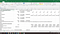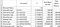# Why the Present Value of a Continuous Compounding Return is an Exponential Function?

The exponential function F(e^-rt) is very commonly used in converting a future value F into the present value P with a continuous compounding return at an annual discount rate r for time period t.

Mathematically, P = F(e^-rt)

However, it is not commonly explained in most journal articles which assume that readers understand the rationale behind. Yet, many students told me that they do not know why, and could not find any simple intuitive explanations (non-mathematical derivations) on web.

A. This article tries to illustrate why an exponential function can convert a future value into the present value. Let’s start at the most simple compound interest formula first.

For a present value P, depositing in a bank at an annual compound interest rate of 7%, then after about 10.25 years (reinvest both principal and interest), the future value will be doubled as follows:

Annually Compounding F1 = P(1+7%)¹⁰.²⁵ = 2P

If we increase the frequency of compounding from once-a-year to twice-a-year (i.e. reinvest every 6 months), then it takes 20.15 half-year, i.e. 10.07 years to double the principal as follows:

Half-yearly Compounding F2 = P(1+7%/2)²⁰.¹⁵ = 2P (i.e. about 10.25 years)

The process can be repeated by increasing the frequency to quarterly, monthly, weekly, daily, hourly, secondly, …., infinitely short period, then the future values becomes:

Quarterly Compounding F3 = P(1+7%/4)³⁹.⁹⁵ = 2P (i.e. about 10.07 years)

Monthly Compounding F4 = P(1+7%/12)¹¹⁹.¹⁷ = 2P (i.e. about 9.93 years)

Weekly Compounding F5 = P(1+7%/52)⁵¹⁵.²⁵ = 2P (i.e. about 9.91 years)

Daily Compounding F6 = P(1+7%/365)³⁶¹³.⁵ = 2P (i.e. about 9.90 years)

Hourly Compounding F7 = P(1+7%/8670)⁸⁶⁷²⁴ = 2P (i.e. about 9.90 years)

Infinitely Short Period of Compounding Fi = P(e⁰.⁰⁷*⁹.⁹⁰) = 2P

The above intuitive illustration shows that a saving scheme with a continuously compounding interest rate could be calculated by an exponential function e^rt, where r is the annual interest rate and t is the number of years of the saving. (Figure 1)

Thus, reversing the process of converting future value back to present value, with a continuous compounding discounting scheme, P = F(e^-rt).Figure 1 Illustration of different frequency of compounding interest schemes to double the principal.

B. Another illustration is to show how the increase of compounding frequency would make the future value conversion factor approaches e = 2.7182818. Take r = 1%, t = 100 years, thus F = exp¹ = 2.7182818. Figure 2 below illustrates how the frequency increase would make F approach e.Figure 2 The frequency increase would make the future value conversion factor approaching e

When it is compounded annually, F1=2.7048138, when it is compounded quarterly, F3=2.7148917, when it is compounded daily, F6 = 2.7182446, when it is compounded hourly, F7 = 2.7182803, they approach towards the exponential function e = 2.7182818. (Figures 2 and 3)

C. A mathematical proof can be found at https://www.math.ubc.ca/~malabika/teaching/ubc/spring11/math105/value.pdf, where the present value of a continuous income stream equals to an integral of the exponential function as shown in the following extract:

Written by